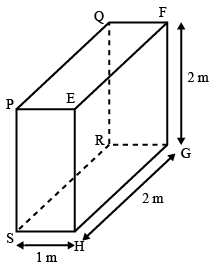# ISRO Scientist or Engineer Refrigeration & Air-Conditioning 2013

Instructions

For the following questions answer them individually

Question 61

# Find the mass of the water vapour per cubic metre of air at temperature 300K and relative humidity 50%. The saturation vapour pressure at 300K is 3.6 kPa and gas constant R = 8.3 $$JK^{-1} mol^{-1}$$Question 62

# For the three dimensional object shown in figure below, five faces are insulated. The sixth face (PQRS), which is not insulated, interacts thermally with the ambient, with a convective heat transfer coefficient of 10 W/m$$^2$$-K. The ambient temperature is $$30^\circ C$$. Heat is uniformly generated inside the object at the rate of 100 W/m$$^3$$. Assuming the face PQRS to be at uniform temperature, its steady state temperature isQuestion 63

# Two walls of thicknesses $$d_1$$ and $$d_2$$ and thermal conductivities $$k_1$$ and $$k_2$$ are in contact. In the steady state, if the temperaturesat the outer surfaces are $$T_1$$ and $$T_2$$ the temperature at the common wall isQuestion 64

# A Carnotcycle refrigerator operates between 250 K and 300 K. Its coefficient of performance (C.O.P.) is:Question 65

# A 3 m$$^3$$ rigid tank contains nitrogen gas at 500 kPa and 300 K. Now heatis transferred to the nitrogen in the tank and the pressure of nitrogen rises to 800 kPa. The work done during this process isQuestion 66

# A solid cylinder (surface 2) is located at the centre of a hollow sphere (surface 1). The diameter of the sphere is 1m, while the cylinder has a diameter and length of 0.5 m each. The radiation configuration factor F11 isQuestion 67

# One kilogram of water at room temperature is broughtinto contact with a high temperature thermal reservoir. The entropy change of the universe isQuestion 68

# For specified limits for the maximum and minimum temperatures, the ideal cycle with the lowest thermal efficiency is:Question 69

# A refrigerator removes heat from a refrigerated space at $$-5^\circ C$$ atarate of 0.35 kJ/s and rejects it to an environment at $$20^\circ C$$. The minimum required power input is:Question 70

# A heat pump is absorbing heat from the cold outdoors at $$5^\circ C$$ and supplying heat to a house at $$22^\circ C$$ at a rate of 18,000 kJ/h. If the power consumed by the heat pump is 2.5 kW, the coefficient of performance (COP) of the heat pump is :OR